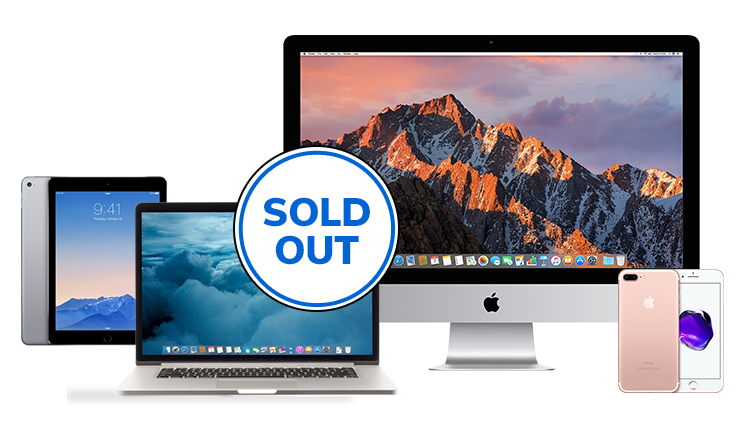• Laptops
Desktops
iPhones & Watches
Accessories & Parts
Refer a Friend, Save \$10
Clearance
Volume
Sell
iPhone 6s & older

Sort By:

Was: \$159.00
Now: \$119.00

Was: \$209.00
Now: \$149.00

Was: \$159.00
Now: \$114.00

Was: \$159.00
Now: \$114.00

Was: \$269.00
Now: \$194.00

Was: \$239.00
Now: \$179.00

Was: \$229.00
Now: \$169.00

Was: \$249.00
Now: \$179.00

Was: \$259.00
Now: \$189.00

Was: \$209.00
Now: \$149.00

Was: \$219.00
Now: \$159.00

Was: \$269.00
Now: \$194.00

Was: \$239.00
Now: \$179.00

Was: \$199.00
Now: \$149.00

Was: \$159.00
Now: \$119.00

Was: \$229.00
Now: \$169.00

Was: \$159.00
Now: \$114.00

Was: \$199.00
Now: \$149.00

Was: \$219.00
Now: \$159.00

Was: \$219.00
Now: \$164.00

Was: \$249.00
Now: \$179.00

Was: \$249.00
Now: \$179.00

Was: \$249.00
Now: \$179.00

Was: \$239.00
Now: \$179.00

Was: \$199.00
Now: \$149.00

Was: \$169.00
Now: \$119.00

Was: \$169.00
Now: \$119.00

Was: \$209.00
Now: \$154.00

Was: \$159.00
Now: \$119.00

Was: \$159.00
Now: \$119.00

Was: \$219.00
Now: \$159.00

Was: \$209.00
Now: \$149.00

Was: \$279.00
Now: \$204.00

Was: \$199.00
Now: \$144.00

Was: \$269.00
Now: \$194.00

Was: \$209.00
Now: \$154.00

Was: \$199.00
Now: \$149.00

Was: \$169.00
Now: \$119.00

Was: \$169.00
Now: \$119.00

Was: \$239.00
Now: \$174.00

Was: \$179.00
Now: \$129.00

Was: \$219.00
Now: \$159.00

Was: \$209.00
Now: \$149.00

Was: \$199.00
Now: \$144.00

Was: \$269.00
Now: \$194.00

Was: \$209.00
Now: \$149.00

\$429.00

Was: \$169.00
Now: \$119.00

\$389.00

Was: \$209.00
Now: \$154.00

Was: \$159.00
Now: \$119.00

Was: \$249.00
Now: \$179.00

Was: \$269.00
Now: \$194.00

Was: \$159.00
Now: \$119.00

Was: \$169.00
Now: \$119.00

Was: \$199.00
Now: \$144.00

Was: \$179.00
Now: \$114.00

Was: \$249.00
Now: \$179.00

Was: \$209.00
Now: \$154.00

Was: \$279.00
Now: \$204.00

Was: \$249.00
Now: \$179.00

Was: \$169.00
Now: \$124.00

Was: \$239.00
Now: \$179.00

Was: \$269.00
Now: \$194.00

\$209.00

Was: \$179.00
Now: \$129.00

Was: \$169.00
Now: \$119.00

Was: \$209.00
Now: \$154.00

Was: \$149.00
Now: \$114.00

Was: \$159.00
Now: \$119.00

Was: \$159.00
Now: \$119.00

Was: \$209.00
Now: \$154.00

Was: \$229.00
Now: \$169.00

Was: \$269.00
Now: \$194.00

Was: \$249.00
Now: \$179.00

Was: \$169.00
Now: \$119.00

Was: \$229.00
Now: \$169.00

Was: \$169.00
Now: \$119.00

Was: \$269.00
Now: \$194.00

Was: \$179.00
Now: \$134.00

\$209.00

Was: \$259.00
Now: \$189.00

Was: \$149.00
Now: \$114.00

\$209.00

\$209.00

\$209.00

\$229.00

Was: \$199.00
Now: \$149.00

Was: \$219.00
Now: \$164.00

\$199.00

Was: \$259.00
Now: \$189.00

Was: \$159.00
Now: \$114.00

Was: \$219.00
Now: \$159.00

Was: \$259.00
Now: \$189.00

Was: \$169.00
Now: \$129.00

Was: \$219.00
Now: \$164.00

Was: \$169.00
Now: \$124.00

Was: \$219.00
Now: \$159.00

Was: \$179.00
Now: \$129.00

#### Apple iPhone 6s (GSM Unlocked) 32GB - Space Gray MN1M2LL/A - Excellent Condition

Was: \$179.00
Now: \$129.00Looks like we sold out!